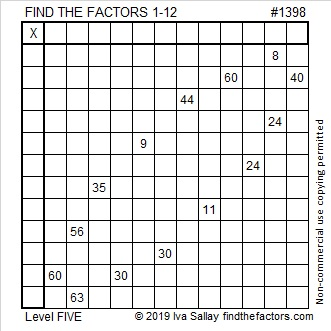# 1398 and Level 5

You might find this puzzle to be a little tricky, but if you always use logic before you write any of the factors, you should succeed!Print the puzzles or type the solution in this excel file: 12 Factors 1389-1403

Here is some information about the number 1398:

• 1398 is a composite number.
• Prime factorization: 1398 = 2 × 3 × 233
• 1398 has no exponents greater than 1 in its prime factorization, so √1398 cannot be simplified.
• The exponents in the prime factorization are 1, 1, and 1. Adding one to each exponent and multiplying we get (1 + 1)(1 + 1)(1 + 1) = 2 × 2 × 2 = 8. Therefore 1398 has exactly 8 factors.
• The factors of 1398 are outlined with their factor pair partners in the graphic below.1398 is the hypotenuse of a Pythagorean triple:
630-1248-1398 which is 6 times (105-208-233)